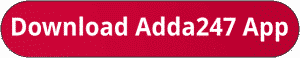Latest Banking jobs   »   IBPS quant

# Quantitative Aptitude Quiz For IBPS Clerk Prelims 2023 – 09th July

Directions (1-5): In each of the following questions, two equations (I) and (II) are given. Solve the equations and mark the correct option:
(a) if x>y
(b) if x≥y
(c) if x<y
(d) if x ≤y
(e) if x = y or no relation can be established between x and y.Directions (6-10): Bar graph given below shows students registered (in lakh) for three different exams in five different years. Study the data carefully and answer the following questions.Q7. Find the ratio of total students registered for all the three exams in 2012 and 2013 together to total students registered for all the three exams in 2014 and 2015 together?
(a) 9 : 17
(b) 2 : 3
(c) 3 : 5
(d) 5 : 8
(e) 10 : 17

Q8. Average number of students registered for MTS exam in all the five years together is how much less/more than average number of students registered for CHSL exam in all the five years together(in lakh)?
(a) 4
(b) 0
(c) 2
(d) 6
(e) 8

Q9. Out of total students registered for all the exams in 2016, 80% appeared for exam. Students appeared in MTS, CGL and CHSL are in the ratio 3 : 3 : 1. Find how much percent of students appeared for exam MTS out of students registered for MTS exam in that year?
(a) 90%
(b) 80%
(c) 60%
(d) 10%
(e) 20%

Q10. In 2013, out of total students registered for all the three exams, 80% appeared out of which only 25% qualified the exams. Find the number of students appeared in exam but disqualified (in Lakh).
(a) 18
(b) 36
(c) 72
(d) 54
(e) 63

Solutions.

## FAQs

### What is the selection process of IBPS Clerk 2023

The selection process of IBPS Clerk includes Prelims and Mains exam

#### Congratulations!Union Budget 2023-24: Free PDF